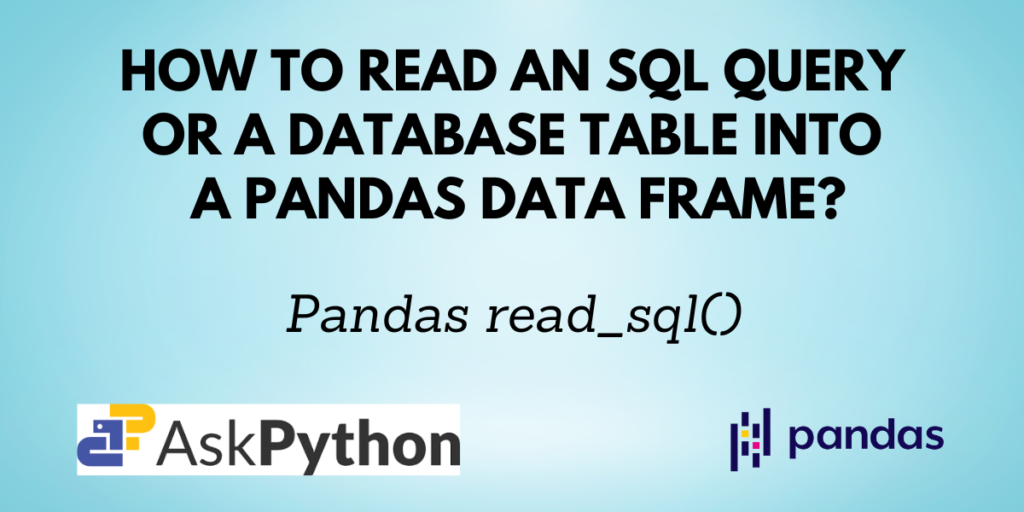For working with datasets, Pandas is the most widely used Python library. You have learned how to use Pandas to read data from files in a variety of formats, including JSON, CSV, Excel, and others, and how to change data in our previous tutorials. One such way is Pandas `read_sql()`, which enables you to read a SQL query or database table into a DataFrame.

You will discover more about the `read_sql()` method for Pandas and how to use it in this article.

Before getting started, you need to have a few things set up on your computer. You need to have Python, Pandas, SQLAlchemy and SQLite and your favorite IDE set up to start coding.

Since SQLAlchemy and SQLite come bundled with the standard Python distribution, you only have to check for Pandas installation. If you do not have it installed by using the command:

```pip install pandas
```

If you are using the Anaconda distribution use the command:

```conda install pandas
```

In this tutorial, we’ll use the file-based database SQLite to set up a connection to a database, add a table, read data from the table, and modify it.

This tutorial expects you to know some of the basic SQL commands.

```pandas.read_sql(sql, con, index_col=None, coerce_float=True, params=None, parse_dates=None, columns=None, chunksize=None)
```

You have now understood the syntax of the `read_sql()` function. Let me now teach you how to work with it.

## Implementing the Pandas read_sql() function

Let us first create a database and a table in it.

```# import the required libraries
from sqlite3 import connect
import pandas as pd

# create a database and connect to it
conn = connect("student.db")
cur = conn.cursor()

# create a table
cur.execute('''CREATE TABLE IF NOT EXISTS STUDENT
([student_id] INTEGER PRIMARY KEY,
[student_name] TEXT,
[course] TEXT,
[marks] INTEGER)
''')

```

The above example can be used to create a database named student.db and then add a table to it using SQL statements. The name of the table is STUDENT and it has 4 columns, namely student_id, student_name, course, and marks.

Next, you need to insert some records into the table. The below code does the job for you.

```# insert rows into the table
cur.execute('''INSERT INTO STUDENT (student_id, student_name, course, marks)
VALUES (1, 'Mark', 'Python', 65),
(2, 'Alice', 'Maths', 55),
(3, 'Bob', 'Linux', 68),
(4, 'Emily', 'English', 50)
''')

# commit the query
conn.commit()
```

4 records have been inserted into the table.

### Reading the table from the database

You can now use the Pandas `read_sql()` function to read the data from the table using SQL queries.

The below example demonstrates how you can load all the data from the table STUDENT and convert it into a Pandas DataFrame.

```# sql query to read all the records
sql_query = pd.read_sql('SELECT * FROM STUDENT', conn)

# convert the SQL table into a pandas dataframe
df = pd.DataFrame(sql_query)

df
```

Output:

### Adding an Index Column to the DataFrame

You can specify the index column for the resulting Pandas DataFrame when implementing the `read_sql()` function.

The below code assigns the column student_id as the index column for the DataFrame.

```# sql query to read all the records
sql_query = pd.read_sql('SELECT * FROM STUDENT', conn, index_col='student_id')

# convert the SQL table into a pandas dataframe
df = pd.DataFrame(sql_query)

df
```

Output:

### Specifying Columns for the output using Pandas read_SQL()

If you do not need all the columns from the database table, you can mention the column names that you need in a list form.

```# sql query to read all the records
sql_query = pd.read_sql('SELECT * FROM STUDENT', conn)

# convert the SQL table into a pandas dataframe
df = pd.DataFrame(sql_query, columns=['student_id', 'student_name'])

df
```

Output:

### Filtering rows using Pandas read_SQL()

Many times, you will not require to read all the rows from the SQL table in the database. So to load only the selected rows, you can filter them using the SQL condition clauses like the WHERE clause.

```# sql query to read all the records having marks>60
sql_query = pd.read_sql('SELECT * FROM STUDENT WHERE marks>60', conn)

# convert the SQL table into a pandas dataframe
df = pd.DataFrame(sql_query)

df
```

Output:

The above code only selects those rows for which the value of marks is greater than 60.

## Conclusion

In this tutorial, you learned about the Pandas read_sql() function which enables the user to read a SQL query into a Pandas DataFrame. You also saw examples that demonstrated how to create a database, add a table to it, display the records from the table, and also how to filter rows for the output using SQL.